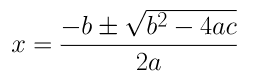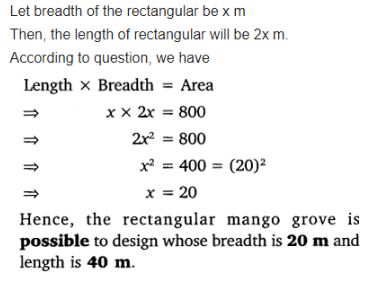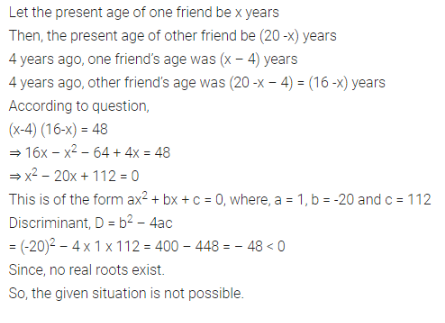• +91 9971497814
• info@interviewmaterial.com

### Related Subjects

Question 1 : Find the nature of the roots of the following quadratic equations. If the real roots exist, find them-

(i) 2x2 –3x + 5 = 0
(ii) 3x2 – 4√3x + 4 = 0
(iii) 2x2 – 6x + 3 = 0

Solution

(i) Given,

2x2 – 3x + 5 = 0

Comparing the equation with ax2 + bx c =0, we get

a = 2, b =-3 and c = 5

We know, Discriminant = b2 –4ac

(– 3)2 – 4 (2) (5) = 9 – 40

= – 31

As you can see, b2 – 4ac <0

Therefore, no real root is possible for thegiven equation, 2x2 – 3x + 5 = 0.

Solution

(ii) 3x2 – 4√3x +4 = 0

Comparing the equation with ax2 + bx c =0, we get

a = 3, b = -4√3 and c =4

We know, Discriminant = b2 –4ac

= (-4√3)– 4(3)(4)

= 48 – 48 = 0

As b2 – 4ac =0,

Real roots exist for the given equation andthey are equal to each other.

Hence the roots will be –b/2a and –b/2a.

b/2= -(-4√3)/2×3 = 4√3/6= 2√3/3 = 2/√3

Therefore, the roots are 2/√3 and2/√3.

Solution

(iii) 2x2 – 6x +3 = 0

Comparing the equation with ax2 + bx c =0, we get

a = 2, b =-6, c = 3

As we know, Discriminant = b2 –4ac

= (-6)2 – 4 (2) (3)

= 36 – 24 = 12

As b2 – 4ac >0,

Therefore, there are distinct real roots existfor this equation, 2x2 – 6x + 3 = 0.= (-(-6) ± √(-62-4(2)(3)) )/ 2(2)

= (6±2√3 )/4

= (3±√3)/2

Therefore the roots for the given equation are(3+√3)/2 and (3-√3)/2

Question 2 : Find the values of k for each of the following quadratic equations, so that they have two equal roots.

(i) 2x2 + kx +3 = 0
(ii) kx (x – 2) + 6 = 0

Solution

(i) 2x2 + kx +3 = 0

Comparing the given equation with ax2 + bx c =0, we get,

a = 2, b =k and c = 3

As we know, Discriminant = b2 –4ac

= (k)2 – 4(2) (3)

k2 – 24

For equal roots, we know,

Discriminant = 0

k2 – 24 = 0

k2 = 24

k = ±√24 = ±2√6

Solution

(ii) kx(x – 2) + 6 = 0

or kx2 – 2kx +6 = 0

Comparing the given equation with ax2 + bx c =0, we get

a = kb =– 2k and c = 6

We know, Discriminant = b2 –4ac

= ( – 2k)2 – 4 (k)(6)

= 4k2 – 24k

For equal roots, we know,

b2 – 4ac = 0

4k2 – 24k =0

4k (k – 6) = 0

Either 4k = 0 or k =6 = 0

k = 0 or k =6

However, if k = 0, then theequation will not have the terms ‘x2‘ and ‘x‘.

Therefore, if this equation has two equalroots, k should be 6 only.

Question 3 : Is it possible to design a rectangular mango grove whose length is twice its breadth, and the area is 800 m2? If so, find its length and breadth.

Answer 3 :Question 4 :
Is the following situation possible? If so, determine their present ages. The sum of the ages of two friends is 20 years. Four years ago, the product of their ages in years was 48.

Answer 4 :Question 5 : Is it possible to design a rectangular park of perimeter 80 and area 400 m2? If so find its length and breadth.

Let the length and breadth of the parkbe and b.

Perimeter of the rectangular park = 2 (l +b) = 80

So, l + b = 40

Or, b = 40 – l

Area of the rectangular park = l×b =l(40 – l) = 40– l= 400

l2   40l +400 = 0, which is a quadratic equation.

Comparing the equation with ax2 + bx c =0, we get

a = 1, b =-40, c = 400

Since, Discriminant = b2 –4ac

=(-40)2 –4 × 400

= 1600 – 1600 = 0

Thus, b2 – 4ac =0

Therefore, this equation has equal real roots.Hence, the situation is possible.

Root of the equation,

l = –b/2a

l = (40)/2(1) =40/2 = 20

Therefore, length of rectangular park, =20 m

And breadth of thepark, = 40 – = 40 – 20 = 20 m.

Todays Deals### Selectedkrishan

Name:
Email:

# Latest News# 9000 interview questions in different categories